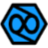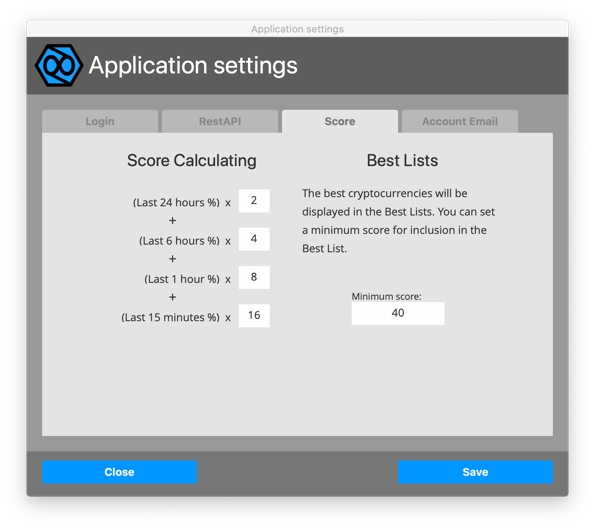# Guide to Crypto Trade Studio

### a knowledge base of Crypto Trade Studio

Home \   Score Calculation

# Score Calculation

The score calculation is the algorithm with which Crypto Trade Studio calculates the "custom" value of each single cryptocurrency.

## How to set up scoring

From the "File" menu select the "Application Settings" itemA general settings mask will appear.

Select the "Score" tab.At this point you will find two sections, one on the left and one on the right.

The left section titled "Score calculation" allows you to determine how the program will calculate the score for each cryptocurrency. There are 4 fields where you can set a value each of which corresponds to the percentage of the last 24 hours, 6 hours, 1 hour, and 15 minutes.

By setting the values 2, 4, 8, 16 the program will calculate:

% growth in the last 24 hours multiplied by 2

plus

% growth in the last 6 hours multiplied by 4

plus

% growth in the last hour multiplied by 8

plus

% growth over the last 15 minutes multiplied by 16

The result obtained will be the score of each cryptocurrency.

In the right section, on the other hand, it is used to indicate to the program which cryptocurrencies will be included in the Best Lists. Here we find only a field to fill in "minimum score" which indicates that if the score of the coin is higher than the one set, that coin will be included in the Best List.